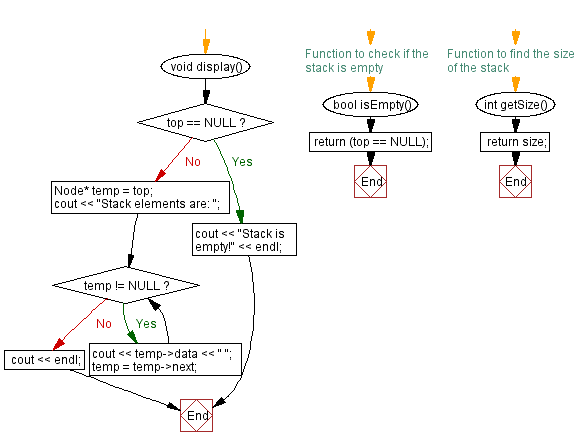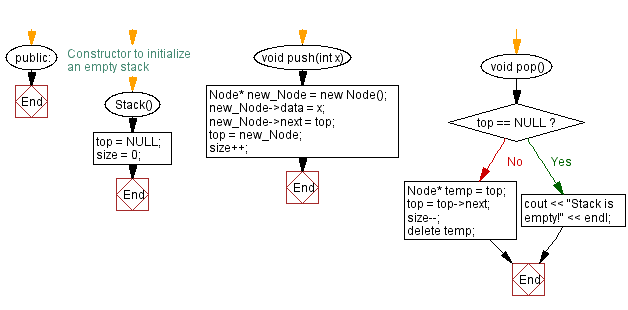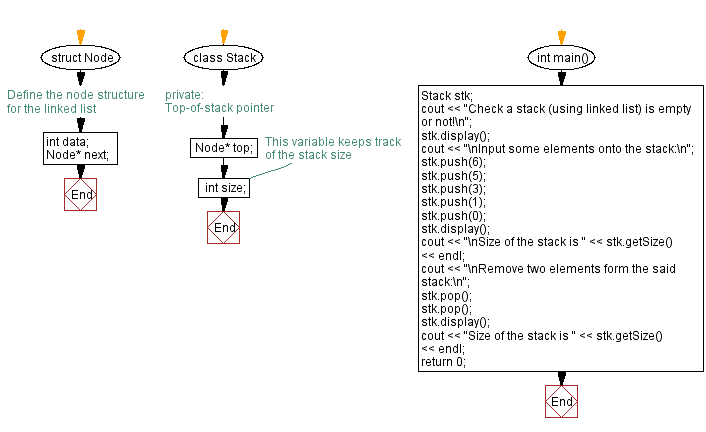﻿ C++ Check the size and empty status of a stack (linked list)

# C++ Stack Exercises: Check the size and empty status of a stack (linked list)

## C++ Stack: Exercise-17 with Solution

Write a C++ program to check a stack's size and whether it is empty or not. The stack is implemented using a linked list.

Test Data:
Check a stack (using linked list) is empty or not!
Stack is empty!
Input some elements onto the stack:
Stack elements are: 0 1 3 5 6
Size of the stack is 5

Sample Solution:

C++ Code:

``````#include <iostream>

using namespace std;

// Define the node structure for the linked list
struct Node {
int data;
Node* next;
};

class Stack {
private:
// Top-of-stack pointer
Node* top;
//This variable keeps track of the stack size
int size;

public:
// Constructor to initialize an empty stack
Stack() {
top = NULL;
size = 0;
}

void push(int x) {
Node* new_Node = new Node();
new_Node->data = x;
new_Node->next = top;
top = new_Node;
size++;
}
void pop() {
if (top == NULL) {
cout << "Stack is empty!" << endl;
return;
}
Node* temp = top;
top = top->next;
size--;
delete temp;
}
void display() {
if (top == NULL) {
cout << "Stack is empty!" << endl;
return;
}
Node* temp = top;
cout << "Stack elements are: ";
while (temp != NULL) {
cout << temp->data << " ";
temp = temp->next;
}
cout << endl;
}
// Function to check if the stack is empty
bool isEmpty() {
return (top == NULL);
}

// Function to find the size of the stack
int getSize() {
return size;
}
};

int main() {
Stack stk;
cout << "Check a stack (using linked list) is empty or not!\n";
stk.display();
cout << "\nInput some elements onto the stack:\n";
stk.push(6);
stk.push(5);
stk.push(3);
stk.push(1);
stk.push(0);
stk.display();
cout << "\nSize of the stack is " << stk.getSize() << endl;
cout << "\nRemove two elements form the said stack:\n";
stk.pop();
stk.pop();
stk.display();
cout << "Size of the stack is " << stk.getSize() << endl;
return 0;
}
``````

Sample Output:

```Check a stack (using linked list) is empty or not!
Stack is empty!

Input some elements onto the stack:
Stack elements are: 0 1 3 5 6

Size of the stack is 5

Remove two elements form the said stack:
Stack elements are: 3 5 6
Size of the stack is 3
```

Flowchart:CPP Code Editor: